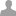## Vector table relocation

All,

We are trying to develop a secondary bootloader which downloads the app code on SPI and jump to it. Everything is working except the interrupts are not getting vectored. we copied the vector table to the RAM and set the VTOR to it. still no change.

We are using LPCXpresso compiler. Is there any sample code to do this on mbed?

Regards, Shankar

You can find source code and the application note for a USB secondary bootloader.

You can take some ideas how you can call your code from sbl_iap.c -> void execute_user_code(void)

Regards Thanassis

Hi Shankar,

####Shankar Telasang wrote:

Everything is working except the interrupts are not getting vectored. we copied the vector table to the RAM and set the VTOR to it. still no change.

Yes, this is exactly what we did in the mbed library:

```#define NVIC_NUM_VECTORS          (16 + 33)     // CORE + MCU Peripherals
#define NVIC_RAM_VECTOR_ADDRESS   (0x10000000)  // Location of vectors in RAM

static volatile uint32_t* vectors = (uint32_t*)NVIC_RAM_VECTOR_ADDRESS;

uint32_t *old_vectors = (uint32_t*)SCB->VTOR;
for (int i=0; i<NVIC_NUM_VECTORS; i++) {
vectors[i] = old_vectors[i];
}
SCB->VTOR = (uint32_t)vectors;
```

HTH, Emilio

Thanks guys.. sorry I was working on different project. Here is my code I am trying. This code has 2 vector tables one that compiler builds and one copy of that. I have 2 ISRs which blink different leds. so I changed each of the vector tables to have different ISR for the timer. It stops working.

1. include "mbed.h"
1. define NVIC_NUM_VECTORS (16 + 33) CORE + MCU Peripherals
2. define NVIC_RAM_VECTOR_ADDRESS1 (0x10000000) Location of vectors in RAM

static volatile uint32_t* vectors1 = (uint32_t*)NVIC_RAM_VECTOR_ADDRESS1;

1. define NVIC_RAM_VECTOR_ADDRESS2 (0x20000000) Location of vectors in RAM

static volatile uint32_t* vectors2 = (uint32_t*)NVIC_RAM_VECTOR_ADDRESS2;

uint32_t *old_vectors; uint32_t *new_vectors;

Ticker flipper;

DigitalOut led1(LED1); DigitalOut led2(LED2); uint32_t count = 0;

void flip1() { led1 = !led1; count++; if(count == 10){ count = 0; SCB->VTOR = (uint32_t)new_vectors; } }

void flip2() { led2 = !led2; count++; if(count == 10){ count = 0; SCB->VTOR = (uint32_t)old_vectors; } }

int main() { led2 = 1; flipper.attach(&flip1, 1.0); the address of the function to be attached (flip) and the interval (2 seconds) old_vectors = (uint32_t*)SCB->VTOR; for (int i=0; i<NVIC_NUM_VECTORS; i++) { new_vectors[i] = old_vectors[i]; } SCB->VTOR = (uint32_t)new_vectors; flipper.attach(&flip2, 0.5); the address of the function to be attached (flip) and the interval (2 seconds) SCB->VTOR = (uint32_t)old_vectors; while(1); }

ignore my prvious post

here is the new one and it do not work.